# (1 point) Definition: The AREA A of the region that lies under the graph of the...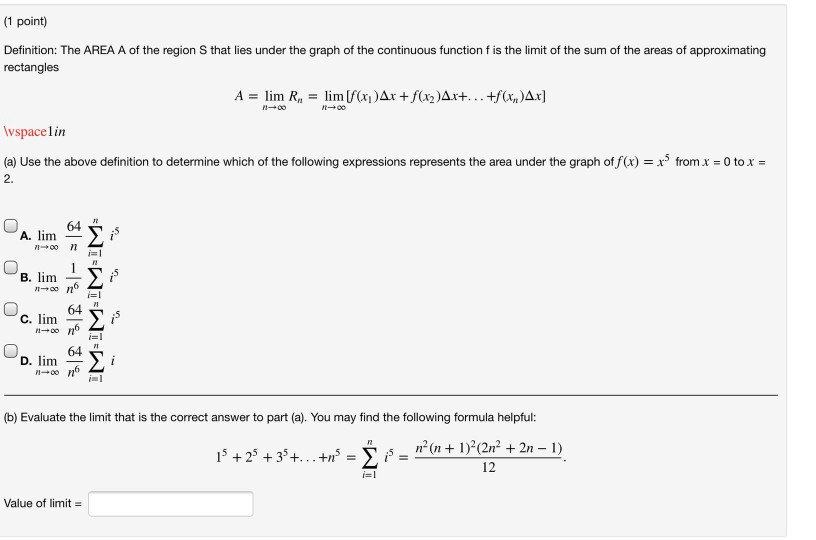(1 point) Definition: The AREA A of the region that lies under the graph of the continuous function f is the limit of the sum of the areas of approximating rectangles A = lim R, = lim [f(x)Ar + f(x2)Ax+... +f(x,y)Ax] 100 Wspacelin (a) Use the above definition to determine which of the following expressions represents the area under the graph of f(x) = x3 from x = 0 to x = 2. 64 A. lim 7100 11 i= B. lim 1100 64 WTWTWTEWI C. lim 00 21 D. lim 11-06 (b) Evaluate the limit that is the correct answer to part (a). You may find the following formula helpful: n-(n + 1 + 2 + 3 +...+ = + 1)(2n2 + 2n-1) 12 n i=1 Value of limit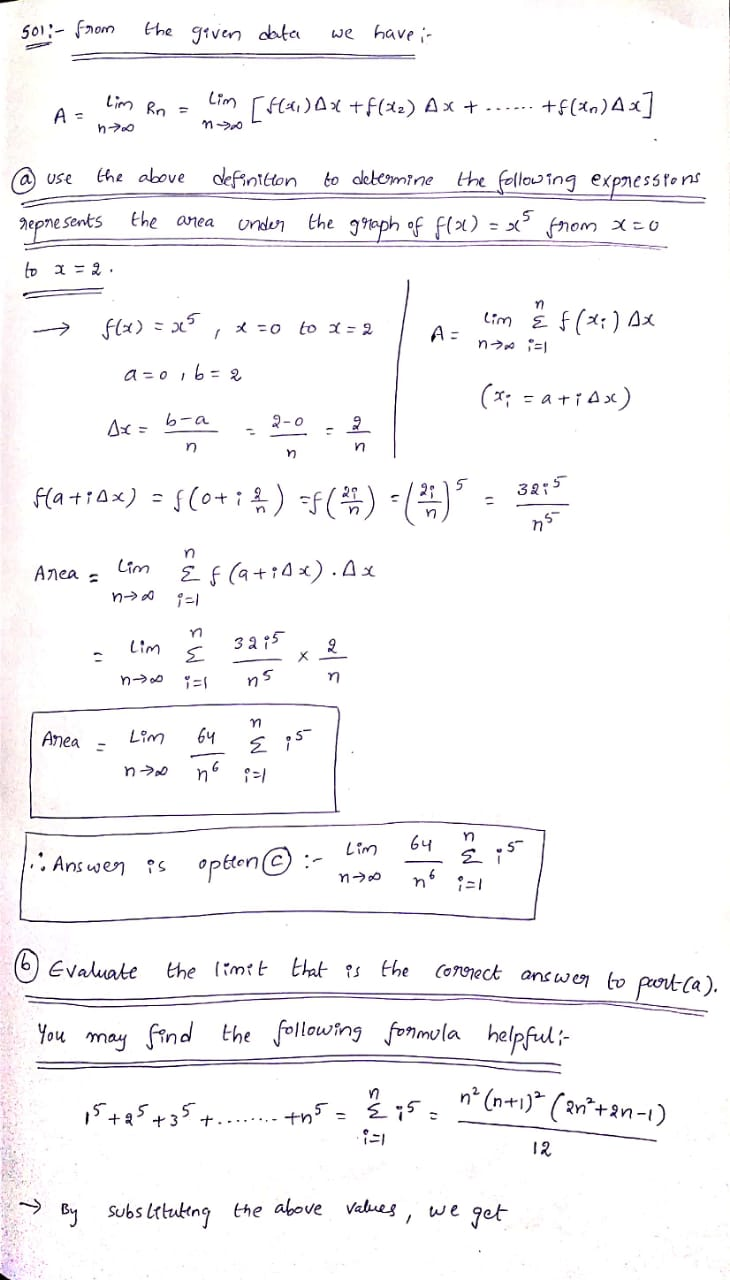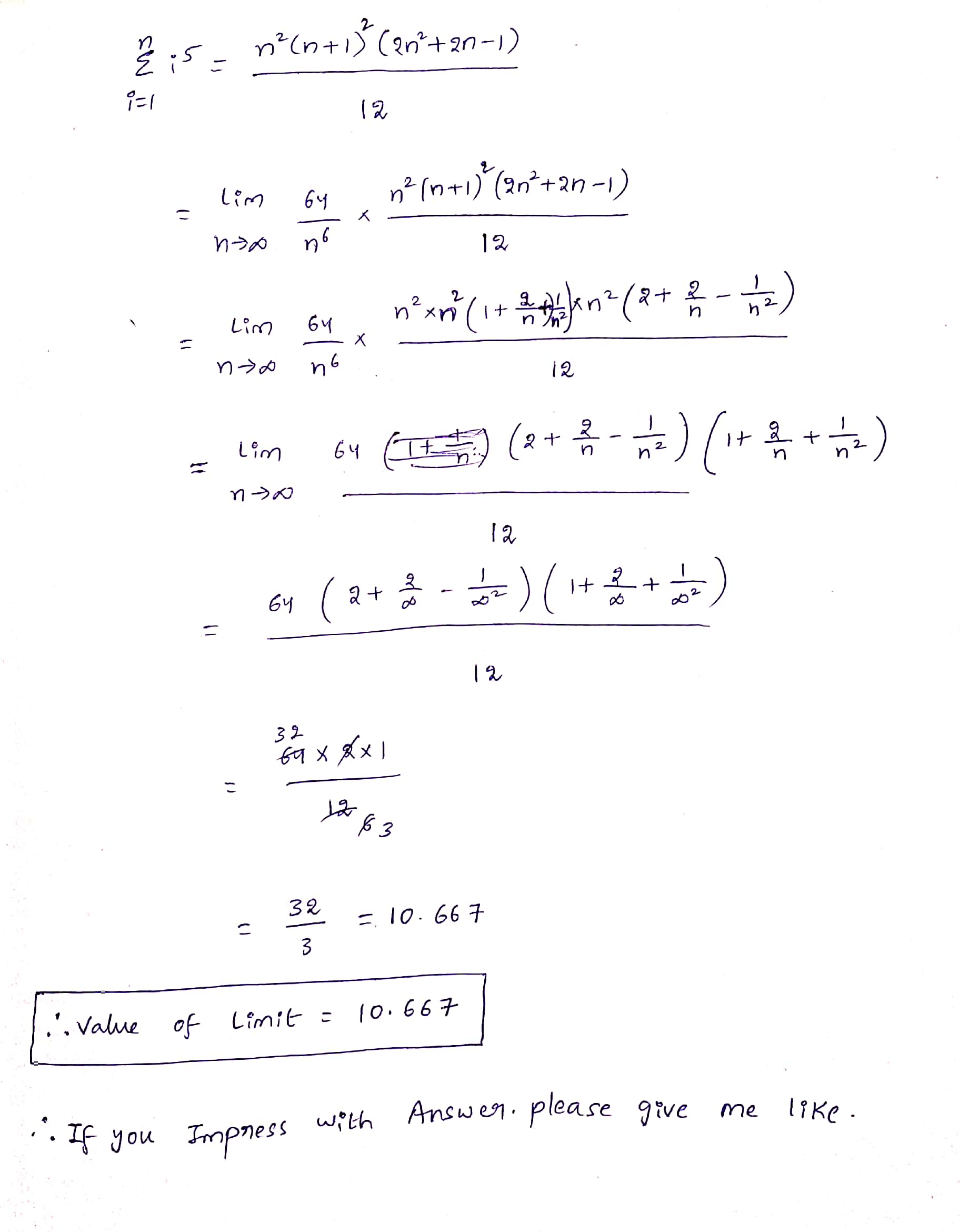#### Earn Coin

Coins can be redeemed for fabulous gifts.

Similar Homework Help Questions
• ### send help for these 4 questions, please show steps Definition: The AREA A of the region...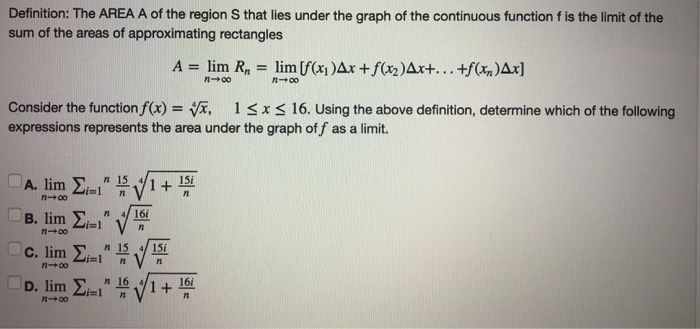send help for these 4 questions, please show steps Definition: The AREA A of the region S that lies under the graph of the continuous function f is the limit of the sum of the areas of approximating rectangles A = lim R, = lim [f(x)Ax +f(x2)Ax+...+f(x)Ax] - 00 Consider the function f(x) = x, 13x < 16. Using the above definition, determine which of the following expressions represents the area under the graph off as a limit. A. lim...

• ### -/2 POINTS SESSCALCET2 5.1.503.XP. The area A of the region that lies under the graph of...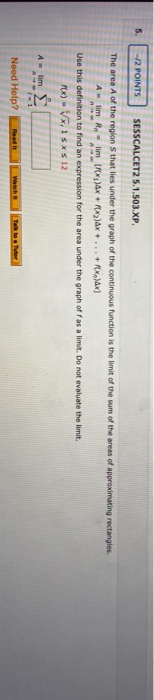-/2 POINTS SESSCALCET2 5.1.503.XP. The area A of the region that lies under the graph of the continuous function is the limit of the sum of the areas of approximating rectangles. lim Rn limf ax + 2)Ax + ... + X)x] Use this definition to find an expression for the area under the graph off as a limit. Do not evaluate the limit. FX) - VX,15* \$ 12 A lim Need Help? Talk to Tutor

• ### The area A of the region S that les under the graph of the continuous fun the areas of approximat...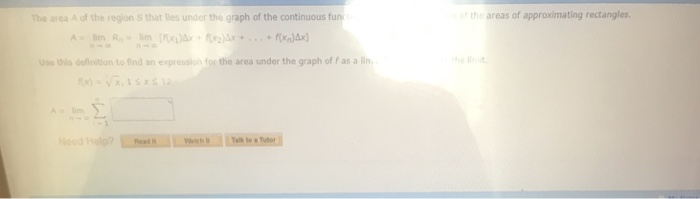The area A of the region S that les under the graph of the continuous fun the areas of approximating rectangles sthis deinition to find an expression for the area under the graph of f as a The area A of the region S that lies under the graph of the continuous function is the limit of the sum of the areas of approximating rectangles Use this definition to find an expression for the area under the graph of f...

• ### Week 1: Problem 21 Previous Problem List Next (1 point) Definition: The AREA A of the...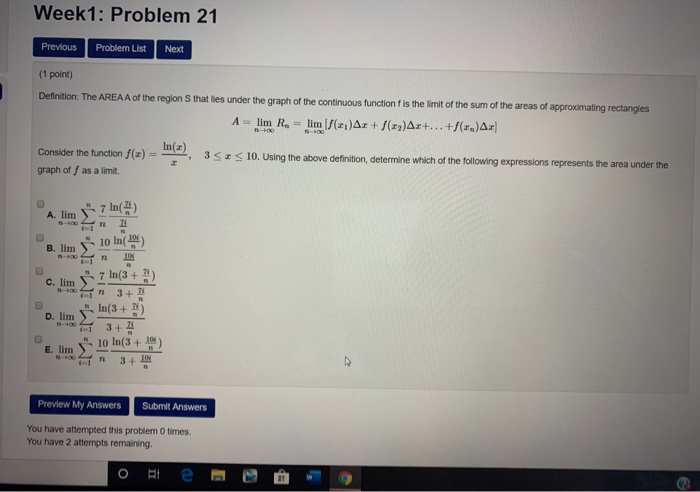Week 1: Problem 21 Previous Problem List Next (1 point) Definition: The AREA A of the region that lies under the graph of the continuous function is the limit of the sum of the areas of approximating rectangles A - lim R. - lim (/(x1)Ar + ()Ar+...+(2.)A: () = 352 10. Using the above definition determine which of the following expressions represents the area under the Consider the function graph off as a limit. A. lim j7 ln() lo in...

• ### (2 points) The area A of the region S that lies under the graph of the...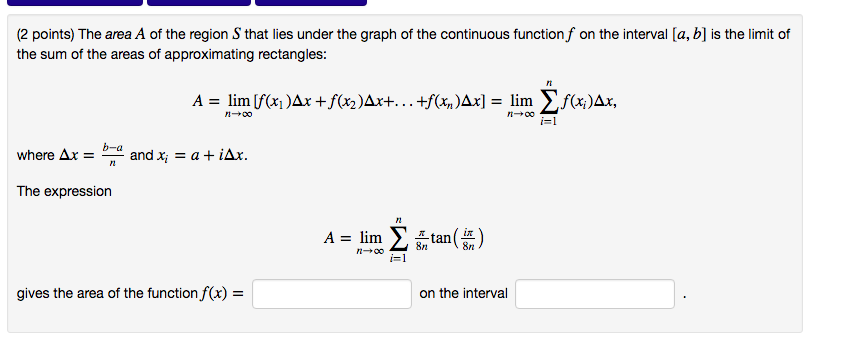(2 points) The area $$A$$ of the region $$S$$ that lies under the graph of the continuous function $$f$$ on the interval $$[a, b]$$ is the limit of the sum of the areas of approximating rectangles:$$A=\lim _{n \rightarrow \infty}\left[f\left(x_{1}\right) \Delta x+f\left(x_{2}\right) \Delta x+\ldots+f\left(x_{n}\right) \Delta x\right]=\lim _{n \rightarrow \infty} \sum_{i=1}^{n} f\left(x_{i}\right) \Delta x$$where $$\Delta x=\frac{b-a}{n}$$ and $$x_{i}=a+i \Delta x$$.The expression$$A=\lim _{n \rightarrow \infty} \sum_{i=1}^{n} \frac{\pi}{8 n} \tan \left(\frac{i \pi}{8 n}\right)$$gives the area of the function $$f(x)=$$ on...

• ### ssignment6: Problem 9 Previous Problem Problem List Next Problem (1 point) The area A of the...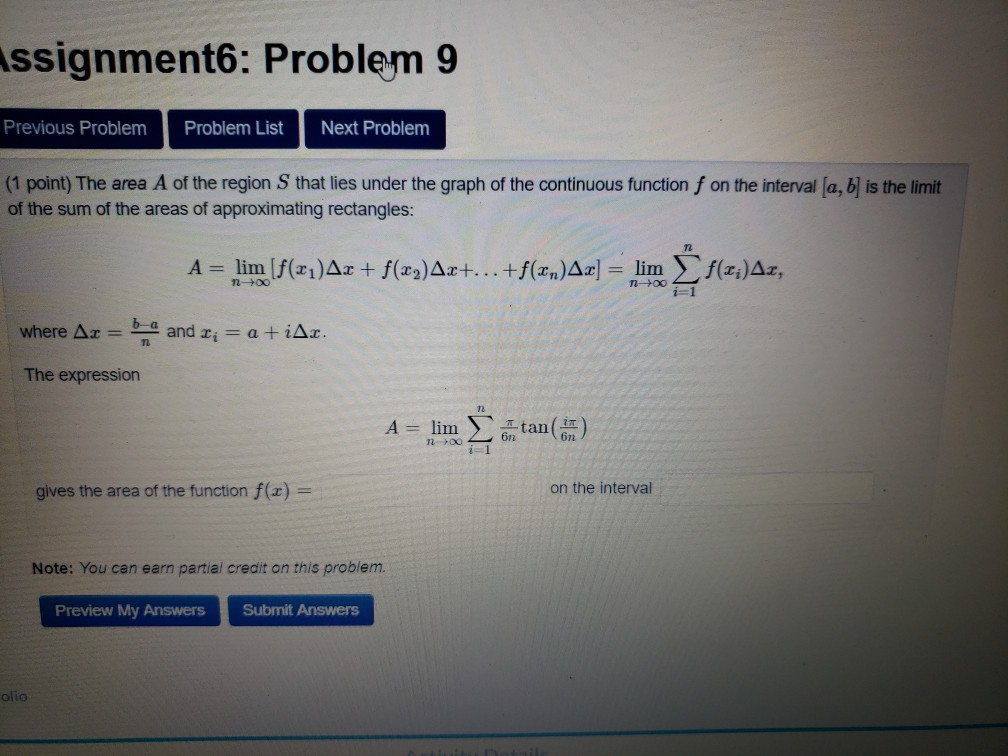ssignment6: Problem 9 Previous Problem Problem List Next Problem (1 point) The area A of the region Sthat lies under the graph of the continuous function f on the interval (a, b) is the limit of the sum of the areas of approximating rectangles: A = lim (f(21)Ar + f(x2)Ax+...+f(xn)Ax] = lim f(x;)Az, n-> ng i=1 where Ax = b and Ti = a +iAr. The expression A = lim Itan(n) 7200 6n2 gives the area of the function f(x)...

• ### Just need the answer to question 6 using the information provided in the block above question...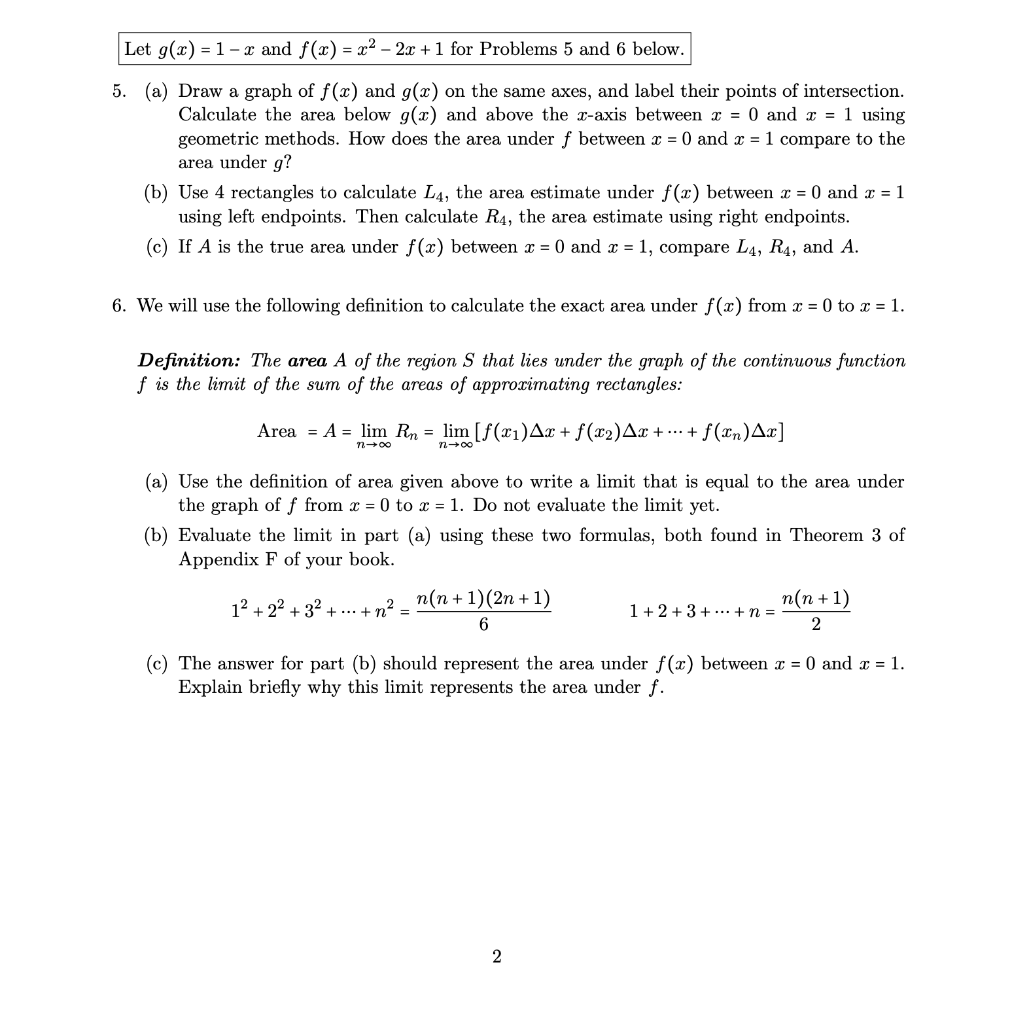Just need the answer to question 6 using the information provided in the block above question 5. Please be clear due to this being a multi-step problem. Thanks    Let g(x) = 1- x and f(x) = x2 - 2x + 1 for Problems 5 and 6 below. 5. (a) Draw a graph of f(x) and g(x) on the same axes, and label their points of intersection. Calculate the area below g(2) and above the z-axis between I = 0 and...

• ### Construct and simplify a sum approximating the area above the x-axis and under the curve y...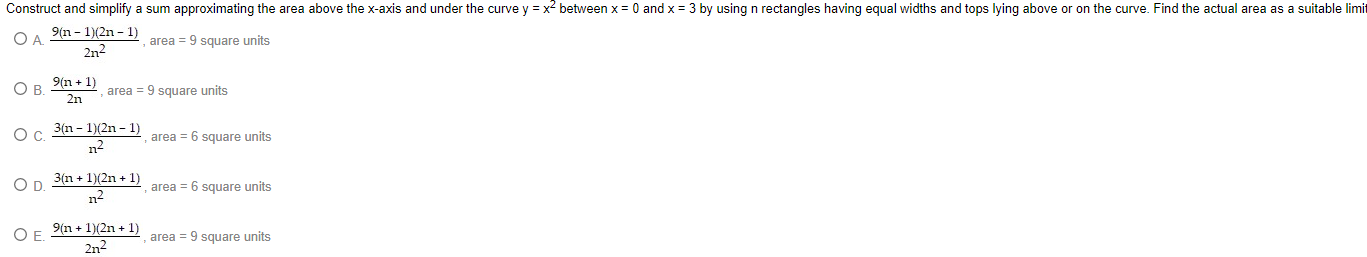Construct and simplify a sum approximating the area above the x-axis and under the curve y = x2 between x = 0 and x = 3 by using n rectangles having equal widths and tops lying above or on the curve. Find the actual area as a suitable limit ОА. 9(n-1)(2n-1) area = 9 square units 2n2 B 9(n + 1) 2n area = 9 square units ос. 3(n-1)(2n-1) n2 area = 6 square units OD 3(n + 1)(2n +...

• ### (1 point) In this problem you will calculate the area between f(x) = x2 and the...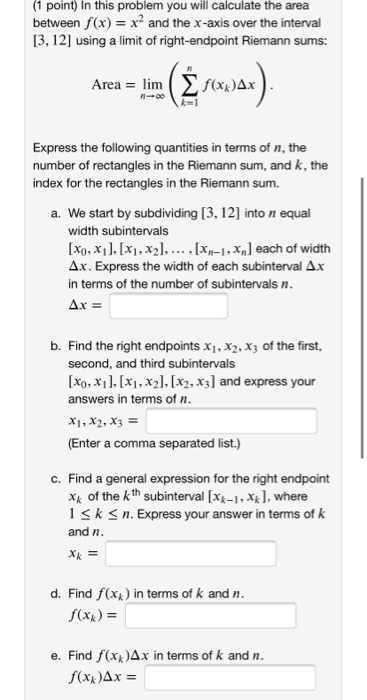(1 point) In this problem you will calculate the area between f(x) = x2 and the x-axis over the interval [3,12] using a limit of right-endpoint Riemann sums: Area = lim ( f(xxAx bir (3 forwar). Express the following quantities in terms of n, the number of rectangles in the Riemann sum, and k, the index for the rectangles in the Riemann sum. a. We start by subdividing [3, 12) into n equal width subintervals [x0, x1], [x1, x2),..., [Xn-1,...

• ### (a) Estimate the area under the graph of f(x) = 2/x from x = 1 to...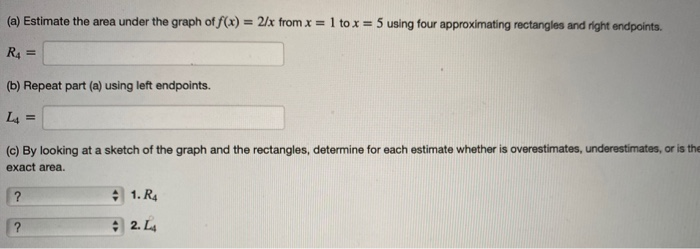(a) Estimate the area under the graph of f(x) = 2/x from x = 1 to x = 5 using four approximating rectangles and right endpoints. | R = (b) Repeat part (a) using left endpoints. L = (c) By looking at a sketch of the graph and the rectangles, determine for each estimate whether is overestimates, underestimates, or is the exact area. ? 1. R4 42. L